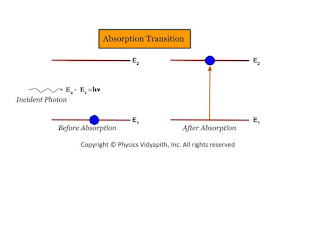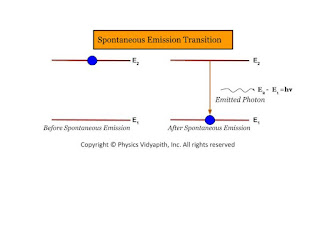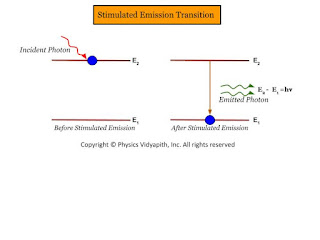### Absorption, Spontaneous Emission and Stimulated Emission of Radiation

Absorption →
When a photon (or light) incident on atoms then atoms absorb the energy from the photon and jump from a lower energy state to a higher energy state. This transition is known as induced absorption or stimulated absorption or simply as absorption.
The process is represented as

$A+h\nu=A^{*}$

Where$A$ → Lower energy state atom$A^{*}$ → Excited or Higher energy state atomAbsorption Transition

If $N_{1}$ and $N_{2}$ is the population of energy $E_{1}$ and $E_{2}$, the number of atoms per unit volume that makes upward transitions from the lower levels to the upper level per second is called the rate of absorption transitions. It is represented by

$R_{abs}=-\frac{dN_{1}}{dt} \qquad(1)$

Where $-\frac{dN_{1}}{dt}$ is rate of decrease of population at the lower energy level $E_{1}$

The rate of absorption transition can also be represented by the rate of the increase of population at the upper energy level $E_{2}$. i.e

$R_{abs}=\frac{dN_{2}}{dt} \qquad(2)$

From equation $(1)$ and equation $(2)$

$R_{abs}=-\frac{dN_{1}}{dt} =\frac{dN_{2}}{dt} \qquad(3)$

The number of absorption transitions occurring within the material at any instant will be proportional to the population in the lower energy level and the number of photons per unit volume in the incident beam. The rate of absorption can be expressed conveniently.

$R_{abs}=B_{12} \rho(v) N_{1} \qquad(4)$

Where$\rho(v)$ → Energy density of incident light$B_{12}$ → Einstien coefficient for induced absorption and it indicates the probability of an induced transition from level 1→2.

At thermal equilibrium, the population in the lower energy state is much larger than that in the higher energy state. Therefore, as light propagates through the medium. These lower energy state atoms gets absorbed.

Spontaneous Emission →
When atoms absorb energy from photons, it goes from lower energy levels to higher energy levels. An excited atom stays at the excited energy level for an average lifetime $\tau_{sp}$. If it is not stimulated by any other agent during its short lifetime, the excited atom undergoes a transition to the lower energy level on its own and gives up the excess energy in the form of a photon. This process is called spontaneous emission.
The process in which an excited atom emits a photon all by itself and without any external impetus is known as spontaneous emission.

The process is represented as

$A^{*} → A+ h \nu \qquad(5)$Spontaneous Emission Transition

The rate of spontaneous transition $R_{sp}$ is given by

$R_{sp}=-\frac{dN_{2}}{dt}= \frac{N_{2}}{\tau_{sp}} \qquad(6)$

The number of emitted photons will be proportional to the population of the excited energy state only and can be expressed as follows

$R_{sp}=A_{21}N_{2}\qquad(7)$

Where $A_{21}$ is known as the Einstein coefficient for spontaneous emission and is a function of frequency and properties of the material. It indicates the probability of spontaneous emission is independent of light energy.

From equation$(6)$ and equation$(7)$

$A_{21}=\frac{1}{\tau_{sp}} \qquad(8)$

Important features of spontaneous emission →
1. The process of spontaneous emission isn't amenable for control from outside.

2. It is essentially probabilistic in nature.

3. The light isn't monochromatic due to various line broadening processes.

4. Due to a lack of directionality, the light spreads in all directions around the source. The intensity of light decreases as the distance from the source increases.

5. The light is incoherent.

6. An atom can radiate into any of the $4\pi$ steradians with any sense of polarization.

Stimulated Emission →
An atom absorbs the energy of a photon, goes from a lower energy level to a high energy level(or excited level). If a photon with appropriate energy interacts with the excited atom, it can trigger the atom to undergo a transition to the lower level and emit another photon. This process is known as stimulated emission.
The process of emission of a photon by an excited state atom through a forced transition occurring under the influence of an external agent is called induced or stimulated emission. The process may be represented as

$A^{*}+h\nu → A+2h\nu \qquad(9)$Stimulated Emission Transition

The rate of stimulated emission of photons is given as by

$R_{st}=B_{21}\rho(v)N_{2} \qquad(10)$

Where $B_{21}$ is Einstein coefficient for stimulated emission

Important features of spontaneous emission →
1. The process of stimulated emission is controlled from the outside.

2. The photon emitted in this process propagates in the same direction as that of the stimulated photon.

3. The emitted photon has exactly the same frequency, phase, and plane of polarization as the incident photon.

4. The light produced in the process is directional, coherence and monochromatics.